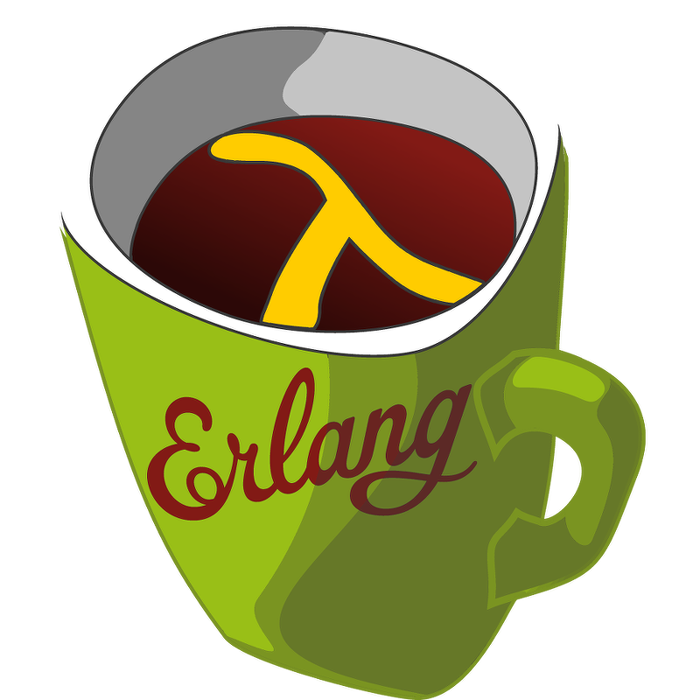This week's LFE Friday was translated with permission from the Erlang Thursday series by Steven Proctor. This week's translator: Robert Virding.

Today's LFE Friday is about lists:flatmap/2.

The trick with `lists:flatmap/2` is working it out what it does, or rather what it does not do. For example it does not take a list of items that are nested arbitrarily deep, and map over the flattened list in the equivalent of this:

``````> (lists:map (lambda (x) (* x x)) (lists:flatten '(1 ((2 (3)) 4))))
(1 4 9 16)``````

In the Erlang docs we see that `lists:flatmap/2` takes a function that takes an item of type `A` and returns a list of items that are of type `B`, and that the second argument to `lists:flatmap/2` was a list of items of type `A`. What this means is best described in the docs by that it behaves as if defined by:

``````(defun flatmap (fun list)
(append (map fun list)))``````

It does the map first and then does the flatten but only one level deep.

``````> (lists:flatmap (match-lambda ((`#(,item ,count))
(lists:duplicate count item)))
'(#(a 1) #(b 2) #(C 3) #(_d_ 4)))
(a b b C C C _d_ _d_ _d_ _d_)``````

And if we pass those values to the "equivalent" behavior of calling map and then calling append on the list returned from map, we see the results are the same.

``````> (lists:append (lists:map (match-lambda ((`#(,item ,count))
(lists:duplicate count item)))
'(#(a 1) #(b 2) #(C 3) #(_d_ 4))))
(a b b C C C _d_ _d_ _d_ _d_)``````

And to further clarify, `lists:flatmap/2` doesn’t even do a flatten on the resulting list, but simply adjoins the lists that were returned from the mapping function. This can be seen below, as we can see there is still an nested list structure in the results, and the resulting list is not only one level deep.

``````> (lists:flatmap (lambda (x) (list x (list x))) '(a b c d))
(a (a) b (b) c (c) d (d))``````

I hope this can save some confusion.

-Proctor, Robert

13 March 2015

tutorials